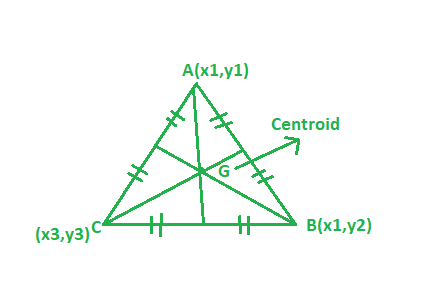Open in App
Not now

# Program to find the Centroid of the triangle

• Last Updated : 28 Aug, 2022

Given the vertices of the triangle. The task is to find the centroid of the triangle:
Examples:

Input: A(1, 2), B(3, -4), C(6, -7)
Output: (3.33, -3)

Input: A(6, 2), B(5, -9), C(2, -7)
Output: (6.5, -9)Approach:Suppose if the vertices of a triangle are (x1, y1) (x2, y2) (x3, y3) then centroid of the triangle can be find from the following formula:## C++

 // CPP program to find the centroid of triangle #include  using namespace std;   // Driver code int main() {     // coordinate of the vertices     float x1 = 1, x2 = 3, x3 = 6;     float y1 = 2, y2 = -4, y3 = -7;       // Formula to calculate centroid     float x = (x1 + x2 + x3) / 3;     float y = (y1 + y2 + y3) / 3;       cout << setprecision(3);     cout << "Centroid = "          << "(" << x << ", " << y << ")";       return 0; }

## Java

 // Java program to find the centroid of triangle import java.util.*; import java.lang.*;   class GFG {       // Driver code public static void main(String args[]) {     // coordinate of the vertices     float x1 = 1, x2 = 3, x3 = 6;     float y1 = 2, y2 = -4, y3 = -7;       // Formula to calculate centroid     float x = (x1 + x2 + x3) / 3;     float y = (y1 + y2 + y3) / 3;       //System.out.print(setprecision(3));     System.out.println("Centroid = "         + "(" + x + ", " + y + ")"); } }   // This code is contributed  // by Akanksha Rai(Abby_akku)

## Python 3

 # Python3 program to find  # the centroid of triangle    # Driver code      if __name__ == "__main__" :       # coordinate of the vertices      x1, x2, x3 = 1, 3, 6     y1, y2, y3 = 2, -4, -7           # Formula to calculate centroid      x = round((x1 + x2 + x3) / 3, 2)     y = round((y1 + y2 + y3) / 3, 2)       print("Centroid =","(",x,",",y,")")   # This code is contributed by ANKITRAI1

## C#

 // C# program to find the  // centroid of triangle using System;   class GFG {       // Driver code static public void Main () {       // coordinate of the vertices     float x1 = 1, x2 = 3, x3 = 6;     float y1 = 2, y2 = -4, y3 = -7;           // Formula to calculate centroid     float x = (x1 + x2 + x3) / 3;     float y = (y1 + y2 + y3) / 3;           //System.out.print(setprecision(3));     Console.Write("Centroid = " +                    "(" + x + ", " + y + ")"); } }   // This code is contributed  // by RaJ

## PHP

 

## Javascript

 

Output:

Centroid = (3.33, -3)

Time complexity: O(1), since there is no loop or recursion.

Auxiliary Space: O(1), since no extra space has been taken.

My Personal Notes arrow_drop_up
Related Articles